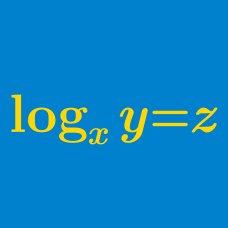Algebra

# Logarithmic Functions: Level 3 Challenges

$\large \log_{\sqrt{x}} \left( \sqrt{x\sqrt{x\sqrt{x\sqrt{x}}}} \right) = \, ?$

• Clarification: $$x$$ is a positive real number and $$x \neq 1.$$

How many digits does the number $$2^{1000}$$ contain?

You are given that $$\log_{10} 2 = 0.3010$$ correct up to 4 decimal places.

$\Large x ^{\log_{10} x } = 100x$

How many real solutions are there to the above equation?

$\Large\begin{cases} \log_x w & = & 24 \\ \log_y w & = & 40 \\ \log_{xyz} w & = & 12 \end{cases}$

If $$x,y,z$$ are real numbers greater than 1 and $$w$$ is a positive number satisfying the system above, then find the value of $$\left (\log_w z \right)^{-1}$$.

Given that

$\log_2(\log_8x)=\log_8(\log_2x),$

find the value of $$(\log_2x)^2$$.

×

Problem Loading...

Note Loading...

Set Loading...